Train

The train passes part of the line for 95 minutes at speed 75 km/h. What speed would have to go in order to shorten the driving time of 20 minutes?

Result

v =  95 km/h

Solution:Leave us a comment of example and its solution (i.e. if it is still somewhat unclear...):Be the first to comment!Next similar examples:

1. Plane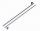On how many parts divide plane 6 parallels?
2. Monthly payments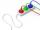Novákovi pay for electricity and monthly pays at the end of the year still bill 34 USD. Total per year pay 618 USD. What were their monthly payments?
3. DoctorsIn the city operates 171 doctors. The city has 128934 citizens. How many citizens are per one doctor?Added together and write as decimal number: LXVII + MLXIV
5. Roman numeralsWrite numbers written in Roman numerals as decimal.
6. To thousands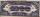Round to thousands following numbers:
7. Compare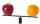Compare with characters >, <, =: 85.57 ? 80.83
8. ProductResult of the product of the numbers 1, 2, 3, 1, 2, 0 is:
9. TriangleProve whether you can construct a triangle ABC, if a=9 cm, b=10 cm, c=4 cm.
10. Roman numerals +Add up the number writtens in Roman numerals. Write the results as a decimal number.
11. MedianThe median of the triangle LMN is away from vertex N 84 cm. Calculate the length of the median, which start at N.
12. Roman numerals 2+Add up the number writtens in Roman numerals. Write the results as a roman numbers.
13. Book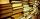Jitka read on holidays book that has 180 pages. In the first week read 45 pages. In the second week she read 15 pages more than the first week. How many pages left to read it yet?
14. Ratio 11Simplify this ratio 10 : 1/4
15. Garden plotCalculate how many meters of fence need to fence the square garden with length and width of 22 meters.
16. Hotel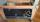The rooms in the mountain hotel are double and triple. Double rooms are 25 and triple are 17 more. How many rooms are there in this hotel?
17. Percentage 1052 shorts and missed 13. Calculate percentage